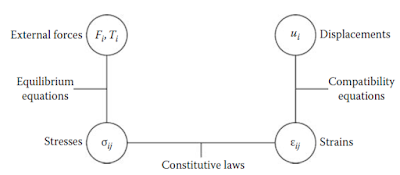# From Science to Engineering

The master builders, the designers, and the constructors of the Gothic cathedrals of the Middle Ages used intuition and experience to develop design rules based on simple force equilibrium and treated the material as rigid. This solution process provided the equivalent of what is now known as the lower-bound theorem of plastic limit analysis. This theorem was not proved until more than 500 years later. Modern lower-bound theorem shows that these design rules are safe. These simple design rules have existed from the earliest times for building Greek temples, Roman aqueducts and arch bridges, and domes and vaults. However, tests on real structures showed that the stresses calculated by designers with these rules could not actually be measured in practice.

Galileo, in the seventeenth century, was the first to introduce recognizably modern science into the calculation of structures; he determined the breaking strength of beams but he was way ahead of his time in engineering application. In the eighteenth century, engineers moved away from his proposed “ultimate load” approach, and, until early in the nineteenth century, a formal philosophy of design had been established: a structure should remain elastic, with a safety factor on stress built into the analysis. It was an era of great advancement and a milestone in structural design but one that placed too much emphasis on the undue safety concern based on elastic response under working loads.

Galileo Galilei (1564–1642) was the first to use mathematics in order to describe the law of nature, which is based on observation from his experiments. Isaac Newton (1642–1727) discovered the basic laws of physics in terms of equilibrium condition (or equation of equilibrium) and equation of motion. Robert Hooke (1635–1703) described, in mathematical form, the material response to stress, which he observed in tests. He stated the linear relationship between stress and strain (Hooke’s law or constitutive law) as a function of a material constant (elasticity modulus or Young’s modulus).

Material continuity without discontinuities or cracks is a logical assumption in solid mechanics. This assumption leads to a mathematical description of geometric relations of a continuous medium known as continuum expressed in the form now known as compatibility conditions. For a continuum, the conditions of equilibrium (physics), constitutive (materials), and continuity (geometry) furnish the three sets of basic equations necessary for solutions in any solid mechanics problem in which structural engineering is one of its applications. In short, the mechanics analysis of a given structural problem or a proposed structural design must involve the mathematical formulation of the following three sets of equations and solutions:

Equilibrium equations or motion reflecting laws of physics (e.g., Newton’s laws)
Constitutive equations or stress–strain relations reflecting material behavior (experiments)
Compatibility equations or kinematical relations reflecting the geometry or continuity of materials (logic)Interrelationship of the three sets of basic field equations

[blogger]

Engineeersdaily

Name

Email *

Message *